+关注继续查看

# 十六、项目：平台游戏

Iain Banks，《The Player of Games》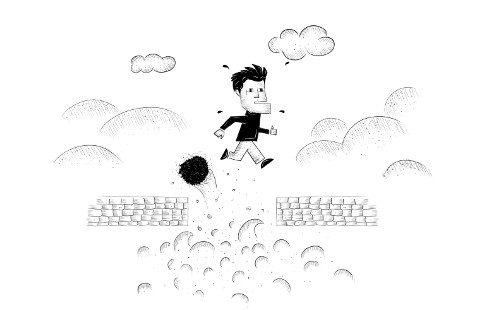## 游戏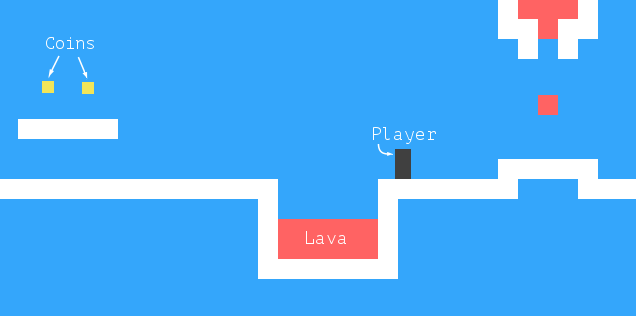## 关卡

var simpleLevelPlan = 
......................
..#................#..
..#..............=.#..
..#.........o.o....#..
..#.@......#####...#..
..#####............#..
......#++++++++++++#..
......##############..
......................;

## 读取关卡

class Level {
constructor(plan) {
let rows = plan.trim().split("\n").map(l => [...l]);
this.height = rows.length;
this.width = rows.length;
this.startActors = [];
this.rows = rows.map((row, y) => {
return row.map((ch, x) => {
let type = levelChars[ch];
if (typeof type == "string") return type;
this.startActors.push(
type.create(new Vec(x, y), ch));
return "empty";
});
});
}
}

trim方法用于移除平面图字符串起始和终止处的空白。这允许我们的示例平面图以换行开始，以便所有行都在彼此的正下方。其余的字符串由换行符拆分，每一行扩展到一个数组中，生成了字符数组。

class State {
constructor(level, actors, status) {
this.level = level;
this.actors = actors;
this.status = status;
}

static start(level) {
return new State(level, level.startActors, "playing");
}

get player() {
return this.actors.find(a => a.type == "player");
}
}

## 角色

type属性包含一个字符串，该字符串指定了角色类型："player""coin"或者"lava"。这在绘制游戏时是有用的，为角色绘制的矩形的外观基于其类型。

class Vec {
constructor(x, y) {
this.x = x; this.y = y;
}
plus(other) {
return new Vec(this.x + other.x, this.y + other.y);
}
times(factor) {
return new Vec(this.x * factor, this.y * factor);
}
}

times方法用给定的数字来缩放向量。当我们需要将速度向量乘时间间隔，来获得那个时间的行走距离时，这就有用了。

class Player {
constructor(pos, speed) {
this.pos = pos;
this.speed = speed;
}

get type() { return "player"; }

static create(pos) {
return new Player(pos.plus(new Vec(0, -0.5)),
new Vec(0, 0));
}
}

Player.prototype.size = new Vec(0.8, 1.5);

size属性对于Player的所有实例都是相同的，因此我们将其存储在原型上，而不是实例本身。我们可以使用一个类似type的读取器，但是每次读取属性时，都会创建并返回一个新的Vec对象，这将是浪费的。（字符串是不可变的，不必在每次求值时重新创建。）

create方法查看Level构造器传递的字符，并创建适当的岩浆角色。

class Lava {
constructor(pos, speed, reset) {
this.pos = pos;
this.speed = speed;
this.reset = reset;
}

get type() { return "lava"; }

static create(pos, ch) {
if (ch == "=") {
return new Lava(pos, new Vec(2, 0));
} else if (ch == "|") {
return new Lava(pos, new Vec(0, 2));
} else if (ch == "v") {
return new Lava(pos, new Vec(0, 3), pos);
}
}
}

Lava.prototype.size = new Vec(1, 1);

Coin对象相对简单，大多时候只需要待在原地即可。但为了使游戏更加有趣，我们让硬币轻微摇晃，也就是会在垂直方向上小幅度来回移动。每个硬币对象都存储了其基本位置，同时使用wobble属性跟踪图像跳动幅度。这两个属性同时决定了硬币的实际位置（存储在pos属性中）。

class Coin {
constructor(pos, basePos, wobble) {
this.pos = pos;
this.basePos = basePos;
this.wobble = wobble;
}

get type() { return "coin"; }

static create(pos) {
let basePos = pos.plus(new Vec(0.2, 0.1));
return new Coin(basePos, basePos,
Math.random() * Math.PI * 2);
}
}

Coin.prototype.size = new Vec(0.6, 0.6);

const levelChars = {
".": "empty", "#": "wall", "+": "lava",
"@": Player, "o": Coin,
"=": Lava, "|": Lava, "v": Lava
};

let simpleLevel = new Level(simpleLevelPlan);
console.log(${simpleLevel.width} by${simpleLevel.height});
// → 22 by 9

## 绘图

function elt(name, attrs, ...children) {
let dom = document.createElement(name);
for (let attr of Object.keys(attrs)) {
dom.setAttribute(attr, attrs[attr]);
}
for (let child of children) {
dom.appendChild(child);
}
return dom;
}

class DOMDisplay {
constructor(parent, level) {
this.dom = elt("div", {class: "game"}, drawGrid(level));
this.actorLayer = null;
parent.appendChild(this.dom);
}

clear() { this.dom.remove(); }
}

const scale = 20;

function drawGrid(level) {
return elt("table", {
class: "background",
style: width: ${level.width * scale}px }, ...level.rows.map(row => elt("tr", {style: height:${scale}px},
...row.map(type => elt("td", {class: type})))
));
}

.background    { background: rgb(52, 166, 251);
table-layout: fixed;
border-spacing: 0;              }
.background td { padding: 0;                     }
.lava          { background: rgb(255, 100, 100); }
.wall          { background: white;              }

function drawActors(actors) {
return elt("div", {}, ...actors.map(actor => {
let rect = elt("div", {class: actor ${actor.type}}); rect.style.width = ${actor.size.x * scale}px;
rect.style.height = ${actor.size.y * scale}px; rect.style.left = ${actor.pos.x * scale}px;
rect.style.top = ${actor.pos.y * scale}px; return rect; })); } 为了赋予一个元素多个类别，我们使用空格来分隔类名。在下面展示的 CSS 代码中，actor类会赋予角色一个绝对坐标。我们将角色的类型名称作为额外的 CSS 类来设置这些元素的颜色。我们并没有再次定义lava类，因为我们可以直接复用前文为岩浆单元格定义的规则。 .actor { position: absolute; } .coin { background: rgb(241, 229, 89); } .player { background: rgb(64, 64, 64); } setState方法用于使显示器显示给定的状态。它首先删除旧角色的图形，如果有的话，然后在他们的新位置上重新绘制角色。试图将 DOM 元素重用于角色，可能很吸引人，但是为了使它有效，我们需要大量的附加记录，来关联角色和 DOM 元素，并确保在角色消失时删除元素。因为游戏中通常只有少数角色，重新绘制它们开销并不大。 DOMDisplay.prototype.setState = function(state) { if (this.actorLayer) this.actorLayer.remove(); this.actorLayer = drawActors(state.actors); this.dom.appendChild(this.actorLayer); this.dom.className = game${state.status};
this.scrollPlayerIntoView(state);
};

.lost .player {
background: rgb(160, 64, 64);
}
.won .player {
box-shadow: -4px -7px 8px white, 4px -7px 8px white;
}

.game {
overflow: hidden;
max-width: 600px;
max-height: 450px;
position: relative;
}

scrollPlayerIntoView方法中，我们找出玩家的位置并更新其包装器元素的滚动坐标。我们可以通过操作元素的scrollLeftscrollTop属性，当玩家接近视口边界时修改滚动坐标。

DOMDisplay.prototype.scrollPlayerIntoView = function(state) {
let width = this.dom.clientWidth;
let height = this.dom.clientHeight;
let margin = width / 3;

// The viewport
let left = this.dom.scrollLeft, right = left + width;
let top = this.dom.scrollTop, bottom = top + height;

let player = state.player;
let center = player.pos.plus(player.size.times(0.5))
.times(scale);

if (center.x < left + margin) {
this.dom.scrollLeft = center.x - margin;
} else if (center.x > right - margin) {
this.dom.scrollLeft = center.x + margin - width;
}
if (center.y < top + margin) {
this.dom.scrollTop = center.y - margin;
} else if (center.y > bottom - margin) {
this.dom.scrollTop = center.y + margin - height;
}
};

<link rel="stylesheet" href="css/game.css">

<script>
let simpleLevel = new Level(simpleLevelPlan);
let display = new DOMDisplay(document.body, simpleLevel);
display.setState(State.start(simpleLevel));
</script>

## 动作与冲突

Level.prototype.touches = function(pos, size, type) {
var xStart = Math.floor(pos.x);
var xEnd = Math.ceil(pos.x + size.x);
var yStart = Math.floor(pos.y);
var yEnd = Math.ceil(pos.y + size.y);

for (var y = yStart; y < yEnd; y++) {
for (var x = xStart; x < xEnd; x++) {
let isOutside = x < 0 || x >= this.width ||
y < 0 || y >= this.height;
let here = isOutside ? "wall" : this.rows[y][x];
if (here == type) return true;
}
}
return false;
};State.prototype.update = function(time, keys) {
let actors = this.actors
.map(actor => actor.update(time, this, keys));
let newState = new State(this.level, actors, this.status);
if (newState.status != "playing") return newState;
let player = newState.player;
if (this.level.touches(player.pos, player.size, "lava")) {
return new State(this.level, actors, "lost");
}
for (let actor of actors) {
if (actor != player && overlap(actor, player)) {
newState = actor.collide(newState);
}
}
return newState;
};

overlap函数检测角色之间的重叠。它需要两个角色对象，当它们触碰时返回true，当它们沿X轴和Y轴重叠时，就是这种情况。

function overlap(actor1, actor2) {
return actor1.pos.x + actor1.size.x > actor2.pos.x &&
actor1.pos.x < actor2.pos.x + actor2.size.x &&
actor1.pos.y + actor1.size.y > actor2.pos.y &&
actor1.pos.y < actor2.pos.y + actor2.size.y;
}

Lava.prototype.collide = function(state) {
return new State(state.level, state.actors, "lost");
};

Coin.prototype.collide = function(state) {
let filtered = state.actors.filter(a => a != this);
let status = state.status;
if (!filtered.some(a => a.type == "coin")) status = "won";
return new State(state.level, filtered, status);
};

## 角色的更新

Lava.prototype.update = function(time, state) {
let newPos = this.pos.plus(this.speed.times(time));
if (!state.level.touches(newPos, this.size, "wall")) {
return new Lava(newPos, this.speed, this.reset);
} else if (this.reset) {
return new Lava(this.reset, this.speed, this.reset);
} else {
return new Lava(this.pos, this.speed.times(-1));
}
};

const wobbleSpeed = 8, wobbleDist = 0.07;

Coin.prototype.update = function(time) {
let wobble = this.wobble + time * wobbleSpeed;
let wobblePos = Math.sin(wobble) * wobbleDist;
return new Coin(this.basePos.plus(new Vec(0, wobblePos)),
this.basePos, wobble);
};

const playerXSpeed = 7;
const gravity = 30;
const jumpSpeed = 17;

Player.prototype.update = function(time, state, keys) {
let xSpeed = 0;
if (keys.ArrowLeft) xSpeed -= playerXSpeed;
if (keys.ArrowRight) xSpeed += playerXSpeed;
let pos = this.pos;
let movedX = pos.plus(new Vec(xSpeed * time, 0));
if (!state.level.touches(movedX, this.size, "wall")) {
pos = movedX;
}

let ySpeed = this.speed.y + time * gravity;
let movedY = pos.plus(new Vec(0, ySpeed * time));
if (!state.level.touches(movedY, this.size, "wall")) {
pos = movedY;
} else if (keys.ArrowUp && ySpeed > 0) {
ySpeed = -jumpSpeed;
} else {
ySpeed = 0;
}
return new Player(pos, new Vec(xSpeed, ySpeed));
};

## 跟踪按键

function trackKeys(keys) {
let down = Object.create(null);
function track(event) {
if (keys.includes(event.key)) {
down[event.key] = event.type == "keydown";
event.preventDefault();
}
}
return down;
}

const arrowKeys =
trackKeys(["ArrowLeft", "ArrowRight", "ArrowUp"]);

## 运行游戏

function runAnimation(frameFunc) {
let lastTime = null;
function frame(time) {
let stop = false;
if (lastTime != null) {
let timeStep = Math.min(time - lastTime, 100) / 1000;
if (frameFunc(timeStep) === false) return;
}
lastTime = time;
requestAnimationFrame(frame);
}
requestAnimationFrame(frame);
}

runLevel函数的接受Level对象和显示对象的构造器，并返回一个PromiserunLevel函数（在document.body中）显示关卡，并使得用户通过该节点操作游戏。当关卡结束时（或胜或负），runLevel会多等一秒（让用户看看发生了什么），清除关卡，并停止动画，如果我们指定了andThen函数，则runLevel会以关卡状态为参数调用该函数。

function runLevel(level, Display) {
let display = new Display(document.body, level);
let state = State.start(level);
let ending = 1;
return new Promise(resolve => {
runAnimation(time => {
state = state.update(time, arrowKeys);
display.setState(state);
if (state.status == "playing") {
return true;
} else if (ending > 0) {
ending -= time;
return true;
} else {
display.clear();
resolve(state.status);
return false;
}
});
});
}

async function runGame(plans, Display) {
for (let level = 0; level < plans.length;) {
let status = await runLevel(new Level(plans[level]),
Display);
if (status == "won") level++;
}
console.log("You've won!");
}

<link rel="stylesheet" href="css/game.css">

<body>
<script>
runGame(GAME_LEVELS, DOMDisplay);
</script>
</body>

## 习题

### 游戏结束

<link rel="stylesheet" href="css/game.css">

<body>
<script>
// The old runGame function. Modify it...
async function runGame(plans, Display) {
for (let level = 0; level < plans.length;) {
let status = await runLevel(new Level(plans[level]),
Display);
if (status == "won") level++;
}
console.log("You've won!");
}
runGame(GAME_LEVELS, DOMDisplay);
</script>
</body>

### 暂停游戏

<link rel="stylesheet" href="css/game.css">

<body>
<script>
// The old runLevel function. Modify this...
function runLevel(level, Display) {
let display = new Display(document.body, level);
let state = State.start(level);
let ending = 1;
return new Promise(resolve => {
runAnimation(time => {
state = state.update(time, arrowKeys);
display.setState(state);
if (state.status == "playing") {
return true;
} else if (ending > 0) {
ending -= time;
return true;
} else {
display.clear();
resolve(state.status);
return false;
}
});
});
}
runGame(GAME_LEVELS, DOMDisplay);
</script>
</body>

### 怪物

<link rel="stylesheet" href="css/game.css">
<style>.monster { background: purple }</style>

<body>
<script>
// Complete the constructor, update, and collide methods
class Monster {
constructor(pos, /* ... */) {}

get type() { return "monster"; }

static create(pos) {
return new Monster(pos.plus(new Vec(0, -1)));
}

update(time, state) {}

collide(state) {}
}

Monster.prototype.size = new Vec(1.2, 2);

levelChars["M"] = Monster;

runLevel(new Level(
..................................
.################################.
.#..............................#.
.#..............................#.
.#..............................#.
.#...........................o..#.
.#..@...........................#.
.##########..............########.
..........#..o..o..o..o..#........
..........#...........M..#........
..........################........
..................................
), DOMDisplay);
</script>
</body>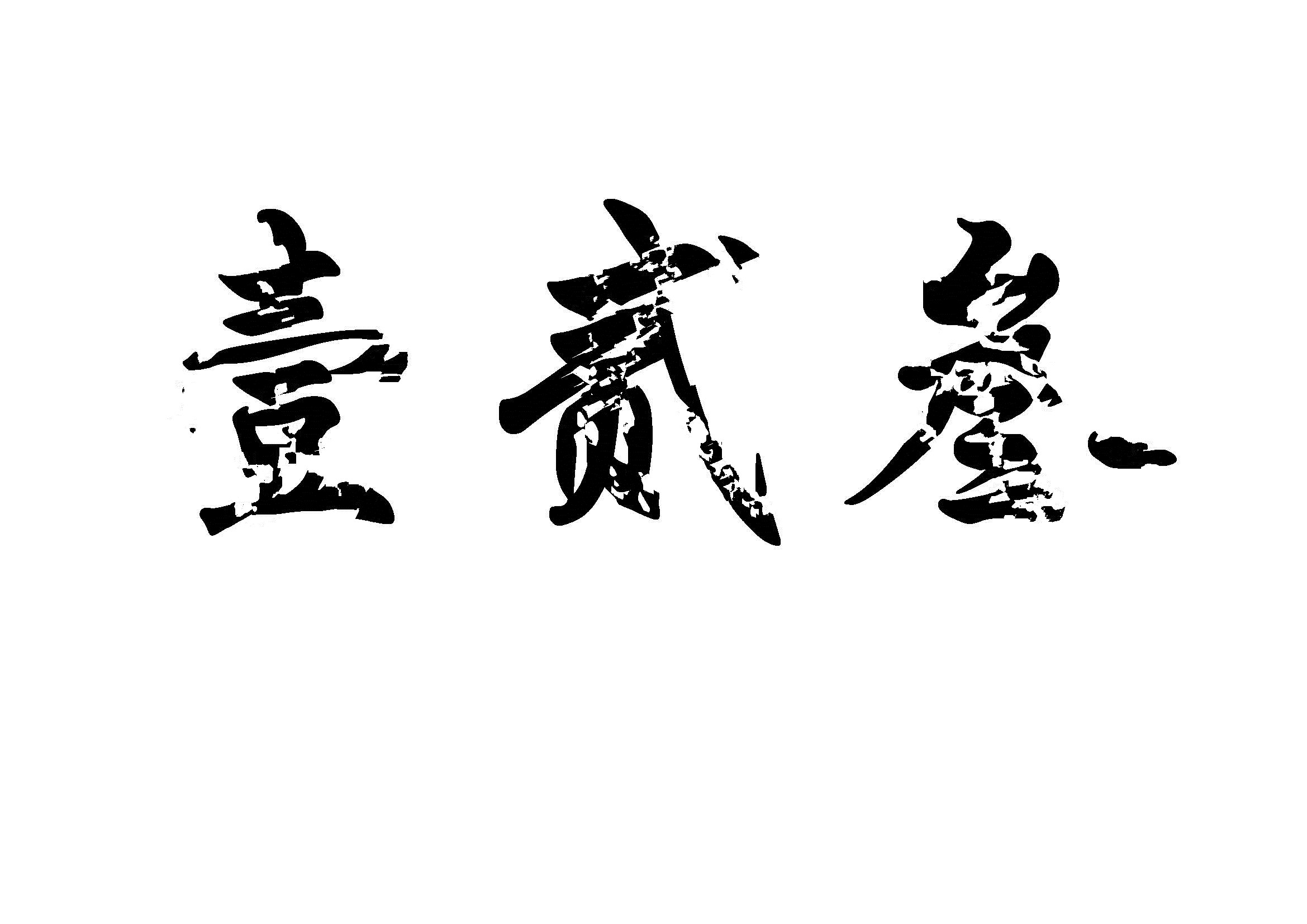JS编程建议——4：注意JavaScript数据类型的特殊性（3）
4：注意JavaScript数据类型的特殊性（3）
1018 0JS编程建议——4：注意JavaScript数据类型的特殊性（1）
4：注意JavaScript数据类型的特殊性（1）
1206 0JS编程建议——4：注意JavaScript数据类型的特殊性（4）
4：注意JavaScript数据类型的特殊性（4）
1087 0JS编程建议——4：注意JavaScript数据类型的特殊性（2）
4：注意JavaScript数据类型的特殊性（2）
953 0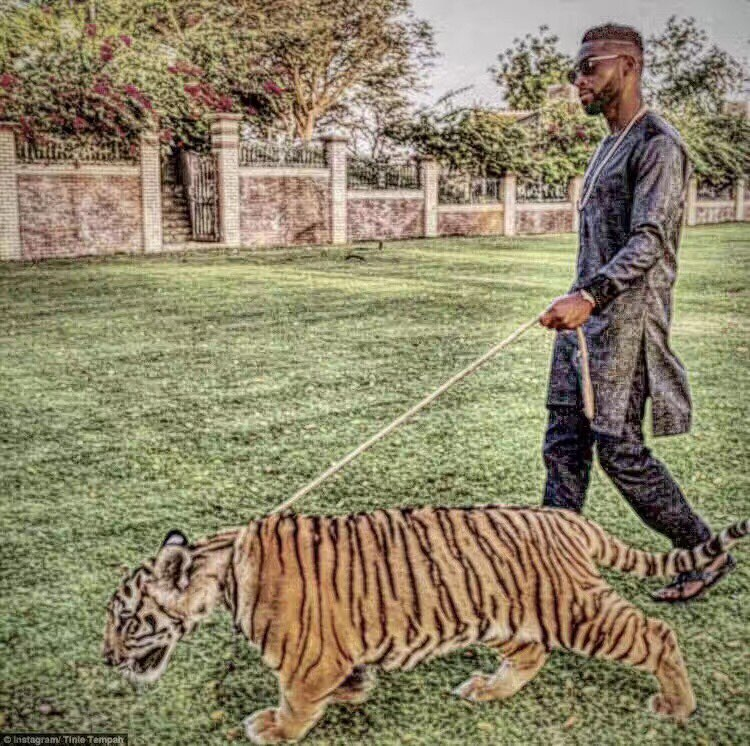1111 0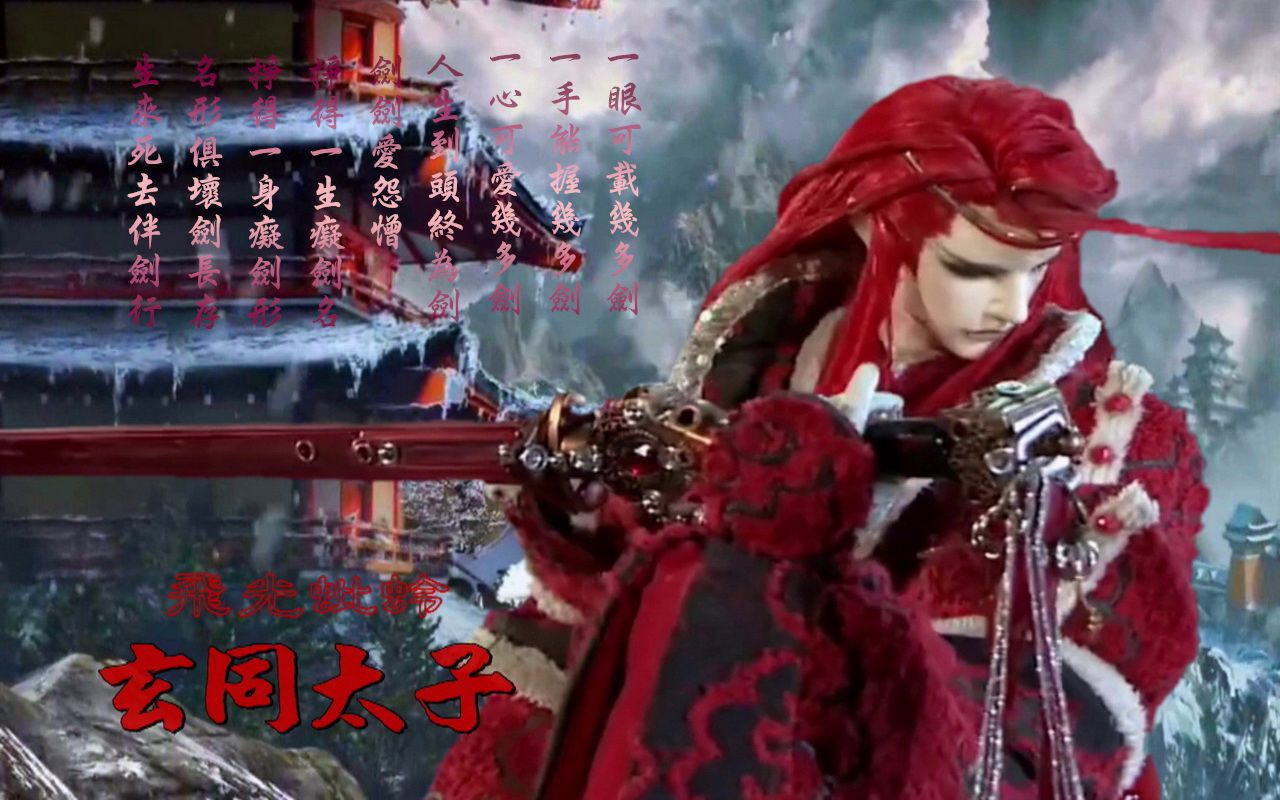1206 0923 01456 0Eclipse中实现JS代码提示功能

1116 0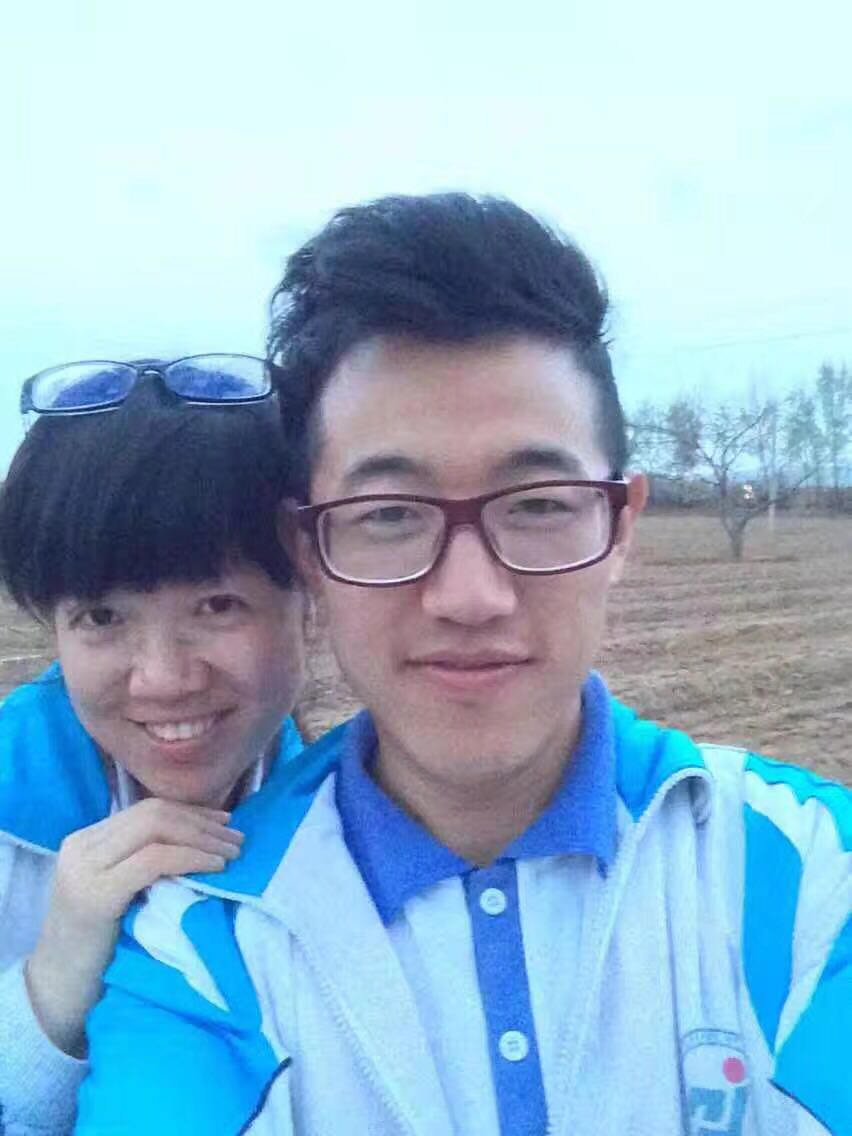JS实现继承的几种方式（转）

979 0+关注
apachecn_飞龙
Github：@wizardforcel 简书：@ApacheCN_飞龙 微博：@龙雀 CSDN：@wizardforcel ApacheCN 官网：apachecn.org 机器学习交流群：629470233
719

0